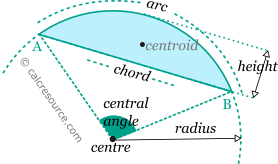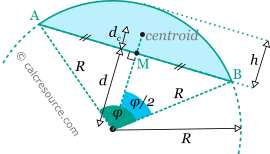Properties of a Circular segment

This tool calculates the basic geometric properties of a circular segment. Enter below the circle radius R and either one of: central angle φ or height h or distance d. Note, that the angle φ can be greater than 180° which represents a segment bigger than the semicircle. In that case distance d is negative and height h is bigger than R. The calculated results will have the same units as your input. Please use consistent units for any input.

 R = φ = deg rad ...or h = ...or d = Geometric properties: φ (rad) = h = d = Area = Perimeter = Arc length = Chord length = dc =Definitions

Geometry

For a circular segment the definitions shown in the following figure are used:For a circular segment with radius R and central angle φ, the chord length LABand its distance d from centre, can be found from the right triangle that occupies half of the region defined by the central angle (see next figure):

The height h of the circular segment h and its arc length L are found easily:The area A and the perimeter P of a circular segment, can be found with these formulas:

where L the arc length and LABthe chord length.

The centroid (center of gravity) of the circular segment is located along the bisector of the central angle φ, and at a distances from the chord equal to:

where d the distance of the chord from the centre.